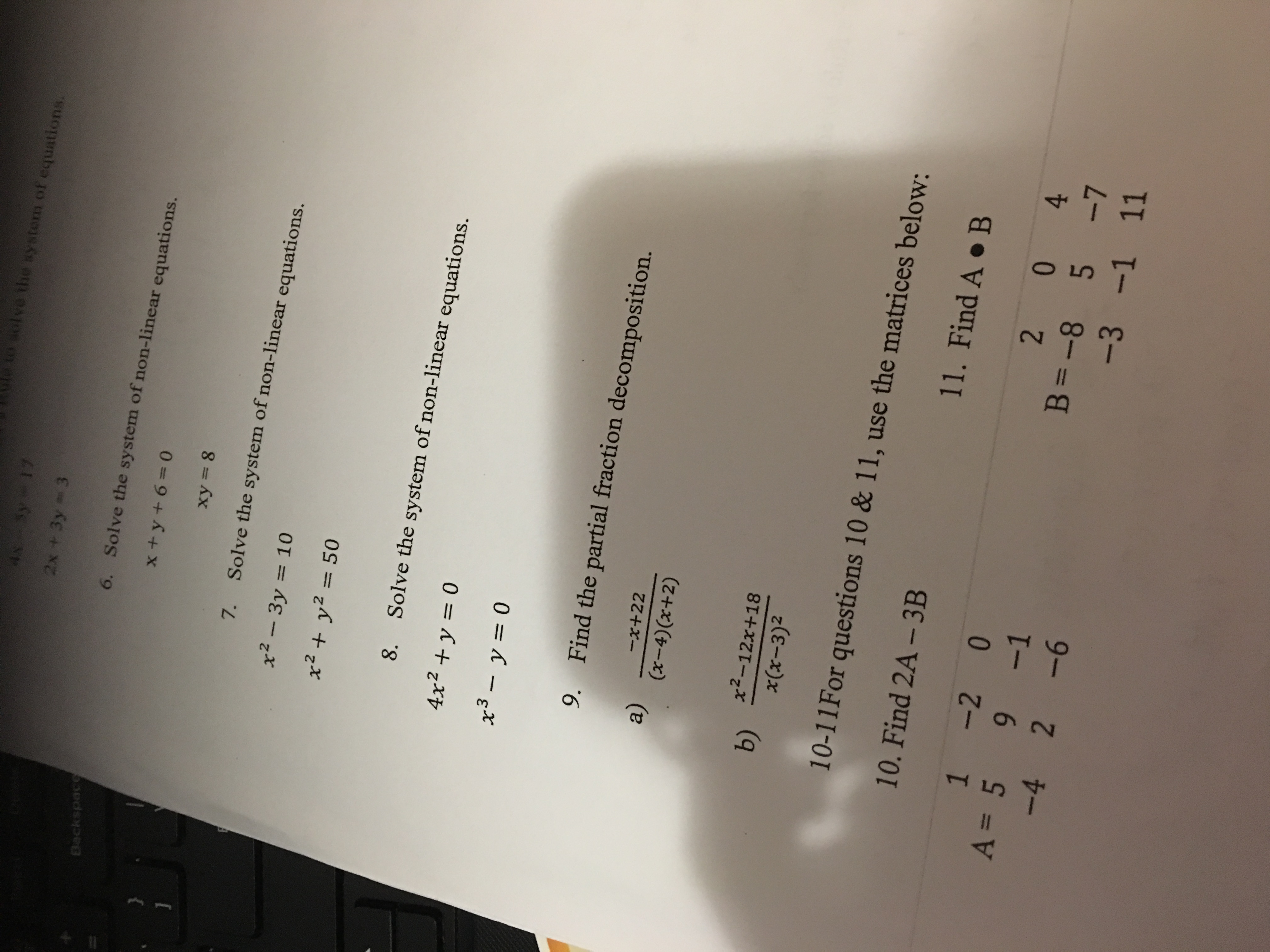# equatio2x +3y 36.Solve the system of non-linear equations.xy- 87. Solve the system of non-linear equations.x2-3y = 10x2+ y2508.Solve the system of non-linear equations.4x2 + y = 09. Find the partial fraction decomposition.a)-x+22(x-4)(r+2)b) 2-12x+1810-11 For questions 10 & 11, use the matrices below:10. Find 2A-3B1 -2 0x(x-3)211 . Find A . ВA=5 9-1-4 2-61B -8 5 71

Question

I need help w/ #’s 7 & 8help_outlineImage Transcriptioncloseequatio 2x +3y 3 6. Solve the system of non-linear equations. xy- 8 7. Solve the system of non-linear equations. x2-3y = 10 x2+ y250 8. Solve the system of non-linear equations. 4x2 + y = 0 9. Find the partial fraction decomposition. a) -x+22 (x-4)(r+2) b) 2-12x+18 10-11 For questions 10 & 11, use the matrices below: 10. Find 2A-3B 1 -2 0 x(x-3)2 11 . Find A . В A=5 9-1 -4 2-6 1 B -8 5 7 1 fullscreen
check_circleExpert Solution
Step 1

7) The system of non-linear equations is

Step 2

Subtract (2) from (1) and eliminate x2 in order to find the value of y as follows.

Step 3

Solve for...

### Want to see the full answer?

See Solution

#### Want to see this answer and more?

Solutions are written by subject experts who are available 24/7. Questions are typically answered within 1 hour*

See Solution
*Response times may vary by subject and question
Tagged in

### Equations and In-equations